# Unicus Olympiads - UCTO PDF Sample Papers for Class 9

Resources:

Class 9 sample paper & practice questions for Unicus Critical Thinking Olympiad (UCTO) PDF Sample Papers are given below. Syllabus for UCTO is also mentioned for these exams. You can refer these sample paper & quiz for preparing for the UCTO exam.Q.1 Q.2 Q.3 Q.4 Q.5 Q.6 Q.7 Q.8 Q.9 Q.10
 Q.1 The diagram given below represents owner, broker and worker. Identify the region which represents all the three i.e. owner, broker and worker: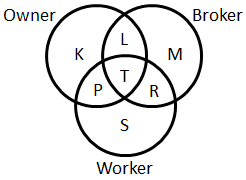a) L b) T c) P d) R
 Q.2 In the following figure, choose the odd one out: a)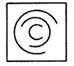b)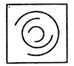c)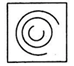d)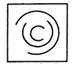Q.3 Starting from the point X. Swati walked 15 m towards West. She turned left and walked 20 m. She then turned left and walked 15 m. After this she turned to her right and walked 12 m. How far and in which direction is now Swati from point X? a) 32 m, South b) 47 m, East c) 42 m, North d) 27 m, South
 Q.4 Which of the following is the same as 'Norway', 'Poland'? a) France b) Rome c) Kenya d) Tokyo
 Q.5 Find the missing term in the series:  6, 22, 86, ?, 1366 a) 340 b) 440 c) 342 d) 240
 Q.6 What would come at the place of the question mark? M A N G O : L N Z B M O F H N P :: R A D I O : ? a) O I D A R b) Q S Z C B C E H J N P c) Q S Z B C H J E N P d) Q S Z B C E H J N P
 Q.7 Read the information carefully and answer the question given below: If '+' means 'x', '-' means '÷', 'x' means '-' and '÷' means '+', then find the value of the given expression:24 + 2 × 4 ÷ 6 - 3 a) 50 b) 46 c) 48 d) 52
 Q.8 Priya scored more than Rohit, Yamini scored as much as Divisha. Lakshita scored less than Mansi. Rohit scored more than Yamini. Mansi scored less than Divisha. Who scored the lowest? a) Mansi b) Yamini c) Lakshita d) Rohit
 Q.9 In the following question, one number is wrong in the series. Find out the number: 1, 3, 8, 19, 42, 89, 184 a) 3 b) 8 c) 19 d) 88
 Q.10 In this question, the relationship between different elements is shown in the statements. The statements are followed by two conclusions. Decide which of the conclusion follows from the statement: Statements: L > M; M > N; N > PConclusions: I. L > P; II. M > P a) Only conclusion I follow b) Only conclusion II follows c) Both conclusions I and II follows d) Neither conclusion I nor II followsSample PDF of Unicus Olympiads - Unicus Critical Thinking Olympiad (UCTO) PDF Sample Papers for Class 9: